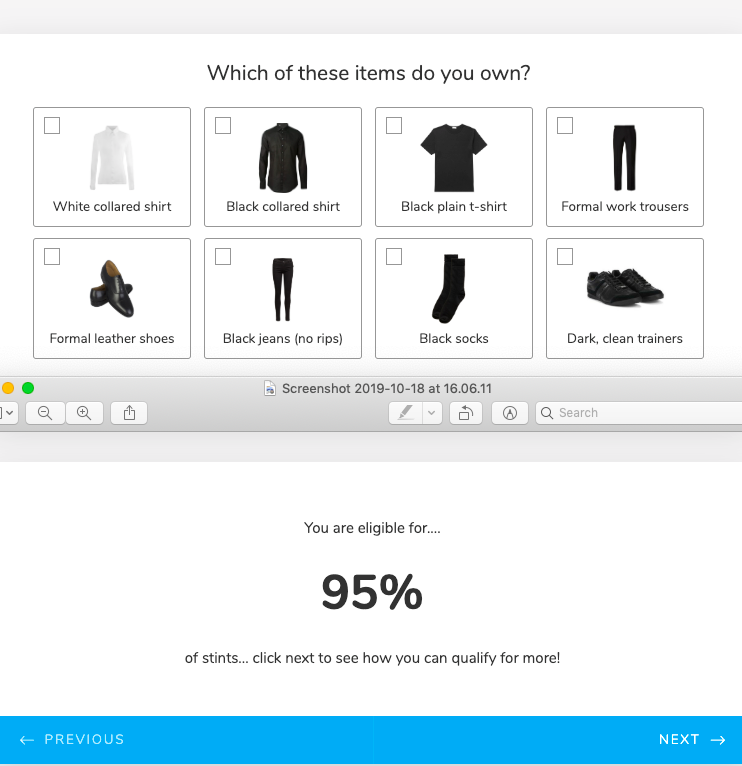# How can I sum up calculations on my form?

•ed16
Asked on October 18, 2019 at 11:08 AM

So I want to create a form where depending on the amount of options selected, a total number is then displayed (e.g. if one is selected = 10, two are selected = 20 etc).•BDAVID
Answered on October 18, 2019 at 11:44 AM

This is possible, all you need to do is to assign a value to each option: https://www.jotform.com/help/301-How-to-Assign-Calculation-ValueAnd add up the total through a Form Calculation widget, example:Let us know if you need more help.